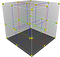# K Dimension TreeA 3D KD Tree model rendered which cuts the plane into two subcells

# What is a K-D Tree ?

A K-D Tree(also called as K-Dimensional Tree) is a binary search tree where data in each node is a K-Dimensional point in space. In short, it is a space partitioning data structure for organising points in a K-Dimensional space.

A non-leaf node in K-D tree divides the space into two parts, called as half-spaces.

Points to the left of this space are represented by the left subtree of that node and points to the right of the space are represented by the right subtree. …## Abhishek Negi

Student , Explorer , Sports Enthusiast